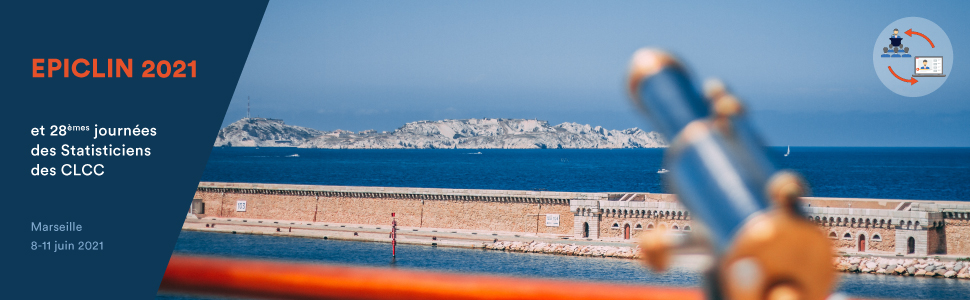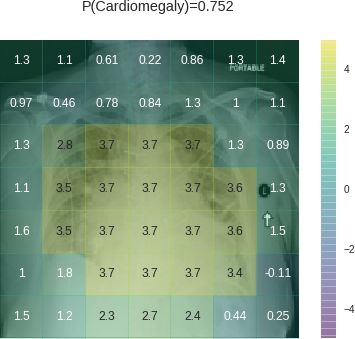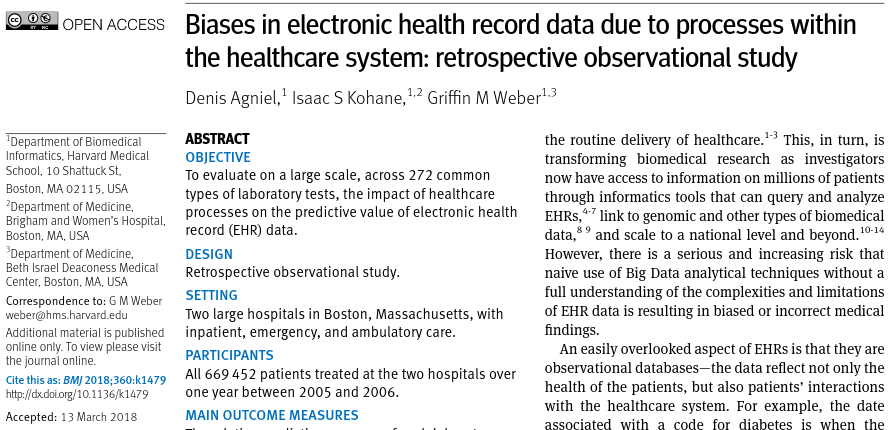EPICLIN2021 / JSCLCC282021-06-09

## Classification

• Classifier: a method providing only categorical predictions
• Classification is a premature decision; a forced choice
• Inconsistent with optimal decision making unless true patient-specific utilities known by analyst
• Best for deterministic outcomes occurring frequently
• Use when probabilities of class membership are all near 0 or 1

## Prediction

• Predictions are separate from decisions & can be used by any decision maker
• When outcome incidence is near 0 or 1 deal with tendencies (probabilities)
• ML too often uses classification and discards observations to get class balance (!)

## Minimum sample Sizes Depending on Goal

• Estimate a single correlation coefficient: n=400 for MOE $$\pm 0.1$$
• Estimate only the intercept in a logistic model: n=96 for MOE $$\pm 0.1$$
• Estimate $$\sigma$$ in linear model: n=70 for MMOE 1.2
• Estimate misclassification probability: n=96 for MOE $$\pm 0.1$$

## Sample Size Requirement, continued

• Select the right variables from a large number: n=$$\infty$$
• Estimate misclassification probability with feature selection or large p: n $$>>$$ 96
• If sample size is not large in comparison with p, it may be insufficient for
• choosing the optimum penalty
• estimating model performance
• estimating variable importance measures

## Sample Size, continued

If n is too small to do something simple, it is too small to do something complex

## Statistical Model

• Probability model for data
• Default assumption of additivity of predictor effects
• Interactions usually must be pre-specified
• Model may be very high dimensional if penalization used
• Very easy to allow for non-linearity
• Suffers from assumptions
• semiparametric models a great help

## Statistical Model, continued

• Regression models are not ML (though do fall under statistical learning)
• Sound of machine learning posing as logistic regression (courtesy of Maarten van Smeden)

When we raise money it’s AI,
when we hire it’s machine learning, and
when we do the work it’s logistic regression

—Juan Miguel Lavista @BDataScientist

## Machine Learning

• No probability model for data
• Algorithmic
• Can deal with high-order interactions
• Allows for non-linearity
• Suffers from lack of assumptions
• Examples: neural net (deep learning), recursive partitioning, random forest, SVM

## ML is Best For …

• Very high S:N settings (visual and sound pattern recognition) and infinite S:N settings (games e.g. Go and chess)
• makes it safe to effectively estimate a large number of parameters
• Also when unlimited training with exact replications are possible (games)
• Very large n
• Outcome is almost deterministic (two identical subjects will have the same outcomes)

## SM is Best For …

• Lower S:N e.g. diagnosing ovarian cancer from clinical signs, symptoms, biomarkers
• Outcome is stochastic
• Lower n

## Where Things Stand

• Clinical researchers are getting less impressed with ML in typical clinical prediction problems
• Multiple comparative studies are showing that gains from ML in low S:N settings is modest

## What is Radiologic Deep Learning Actually Learning?John Zech medium.com/@jrzech

## Test Ordering vs. Test Results